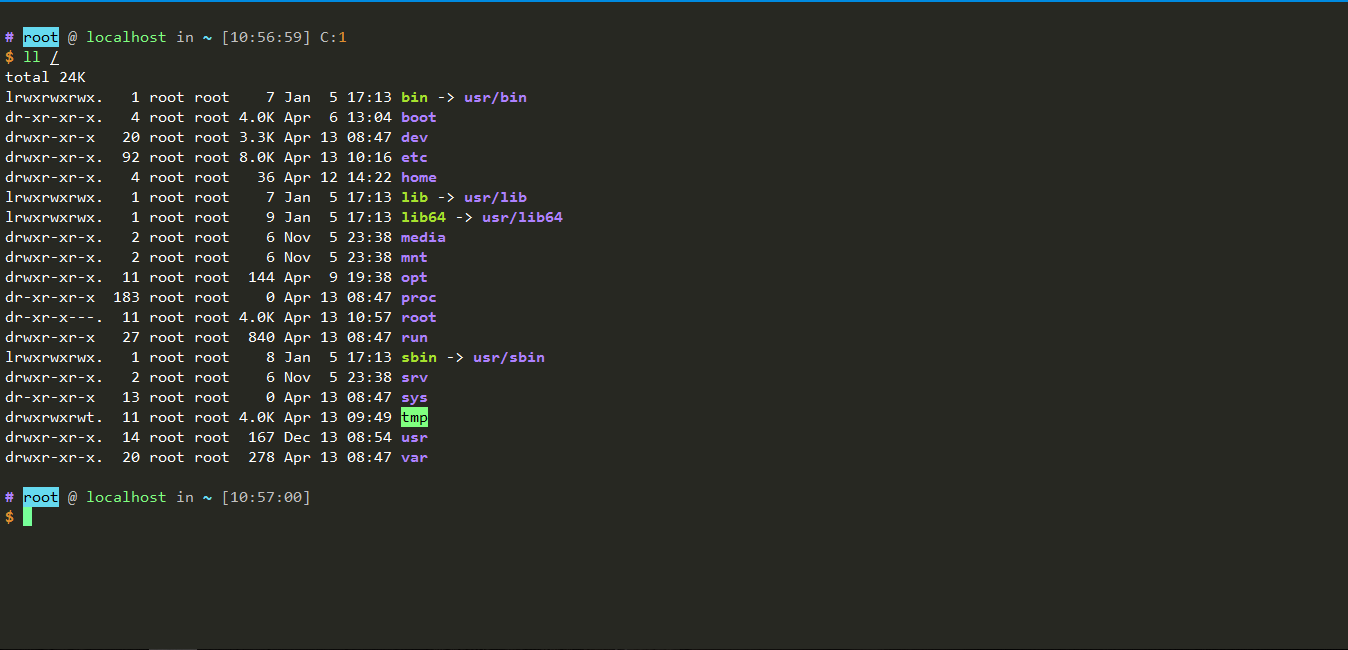xshell5 自带的主题都不是很好看，看久了比较伤眼睛。不过，最近找到了一个 monokai 主题，加上我的 vim 也是 monokai 配色，真是完美啊。

### 主题文件

``````[monokai]
text=ffffff
cyan(bold)=a6e22e
text(bold)=ffffff
magenta=f92672
green=80ff80
green(bold)=80ff80
background=272822
cyan=a6e22e
red(bold)=de8e30
yellow=66d9ef
magenta(bold)=f92672
yellow(bold)=66d9ef
red=de8e30
white=c0c0c0
blue(bold)=ae81ff
white(bold)=ffffff
black=000000
blue=ae81ff
black(bold)=000000
[Names]
name0=monokai
count=1
``````

### 应用主题